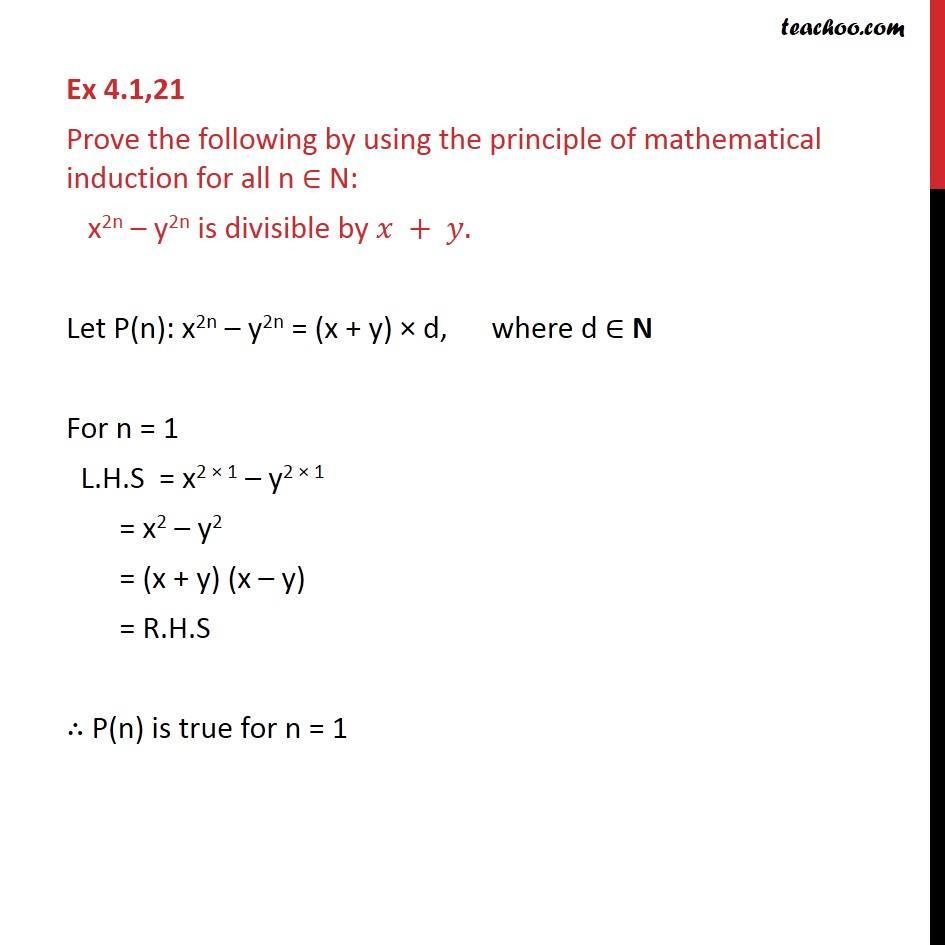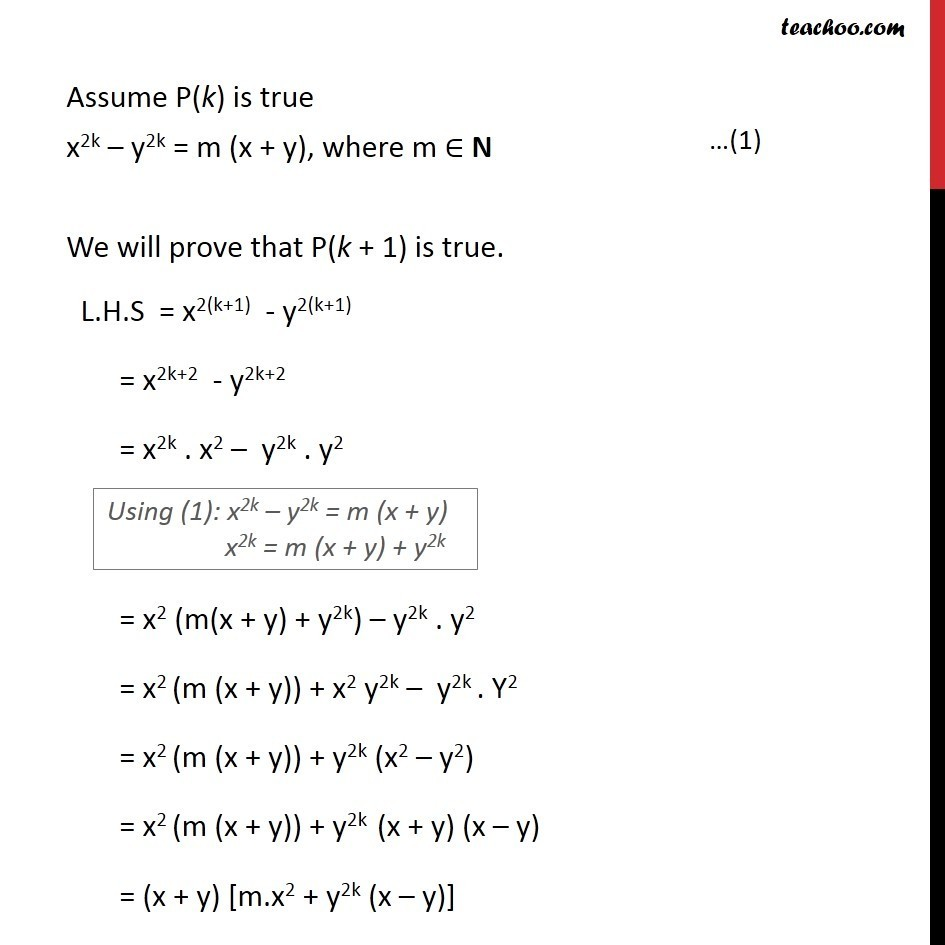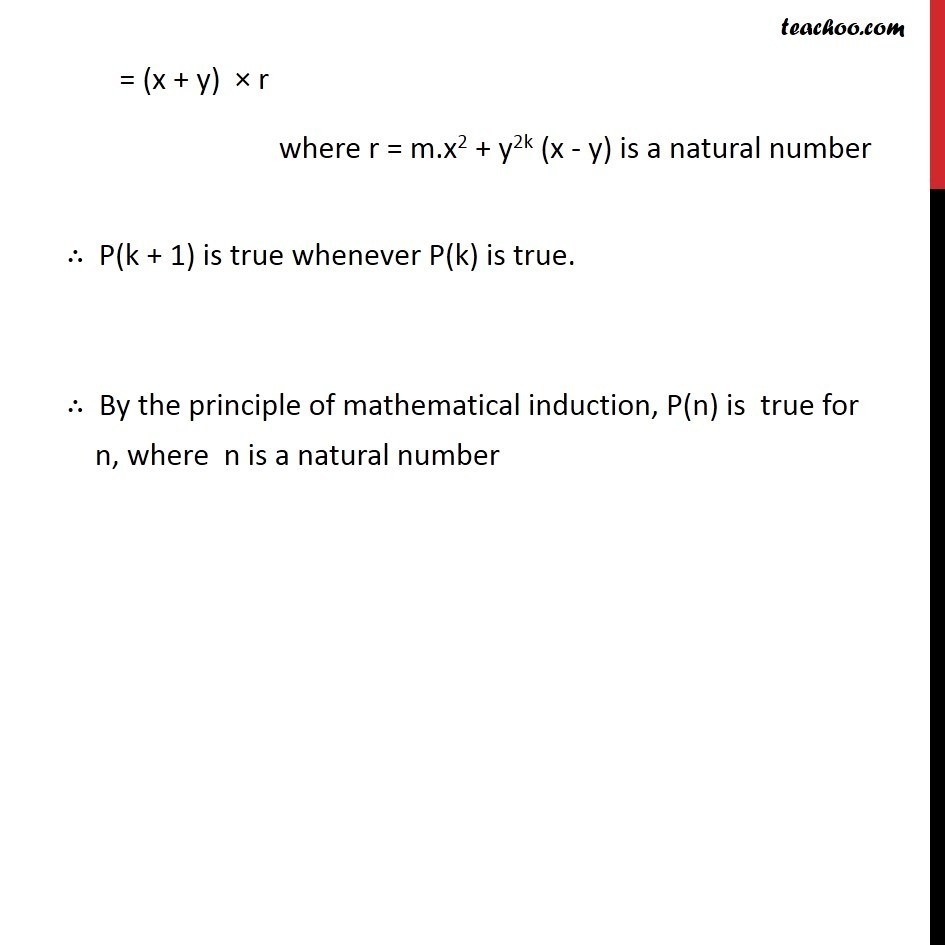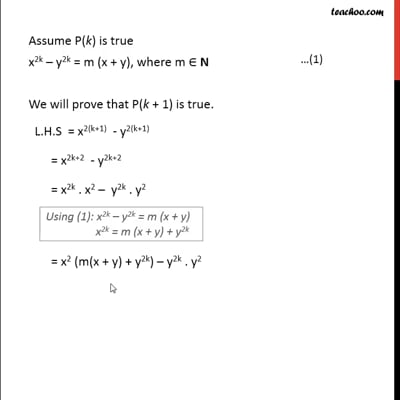Ex 4.1

Chapter 4 Class 11 Mathematical Induction (Deleted)
Serial order wiseThis video is only available for Teachoo black users

### Transcript

Ex 4.1,21 Prove the following by using the principle of mathematical induction for all n ∈ N: x2n – y2n is divisible by 𝑥 + 𝑦. Let P(n): x2n – y2n = (x + y) × d, where d ∈ N For n = 1 L.H.S = x2 × 1 – y2 × 1 = x2 – y2 = (x + y) (x – y) = R.H.S ∴ P(n) is true for n = 1 Assume P(k) is true x2k – y2k = m (x + y), where m ∈ N We will prove that P(k + 1) is true. L.H.S = x2(k+1) - y2(k+1) = x2k+2 - y2k+2 = x2k . x2 – y2k . y2 = x2 (m(x + y) + y2k) – y2k . y2 = x2 (m (x + y)) + x2 y2k – y2k . Y2 = x2 (m (x + y)) + y2k (x2 – y2) = x2 (m (x + y)) + y2k (x + y) (x – y) = (x + y) [m.x2 + y2k (x – y)] = (x + y) × r where r = m.x2 + y2k (x - y) is a natural number ∴ P(k + 1) is true whenever P(k) is true. ∴ By the principle of mathematical induction, P(n) is true for n, where n is a natural number